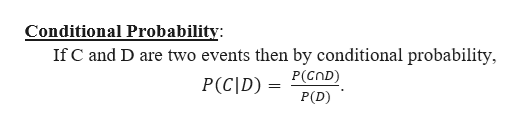# Let C and D be events such that​ Pr[C] =0.70.7​, Pr[D] =0.50.5​, andPr​ [C ∪​ D] =0.90.9.Find...Pr[C |​ D].Pr[D |​ C].

Question

Let C and D be events such that​ Pr[C] =0.70.7​, Pr[D] =0.50.5​, and

Pr​ [C ∪​ D] =0.90.9.

Find...

Pr[C |​ D].

Pr[D |​ C].

check_circleExpert Solution
Step 1

We are given that C and D be events such that​ P(C) = 0.7​, P(D) = 0.5 and P(C ∪​ D) = 0.9 .

Before ...help_outlineImage TranscriptioncloseConditional Probability: If C and D are two events then by conditional probability P(C]D) = P(COD) P(D) fullscreen

### Want to see the full answer?

See Solution

#### Want to see this answer and more?

Solutions are written by subject experts who are available 24/7. Questions are typically answered within 1 hour*

See Solution
*Response times may vary by subject and question
Tagged in

### Basic Probability